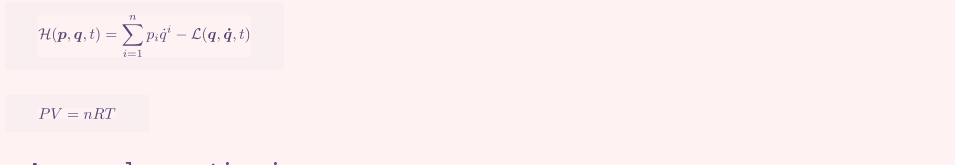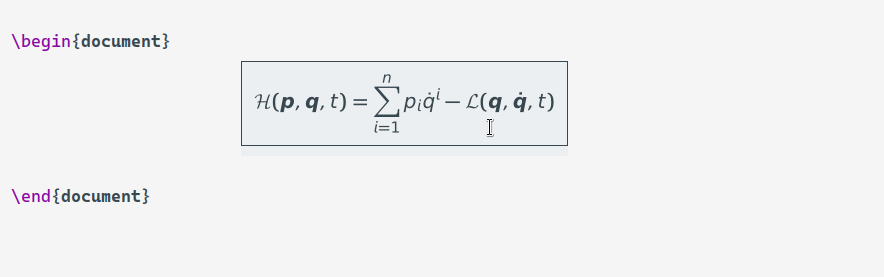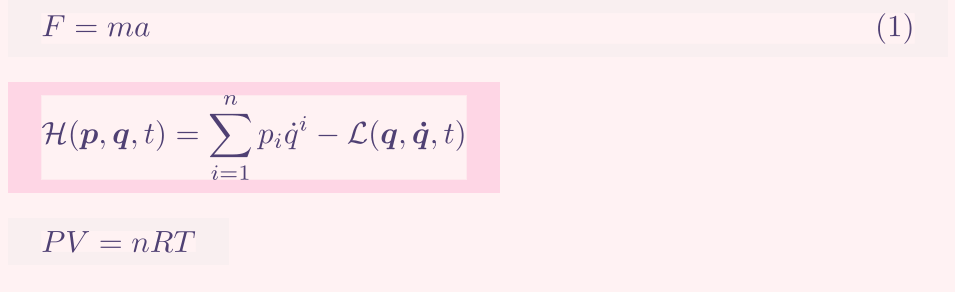# 【分享】在 org-mode 和 latex-mode 中美化公式预览

latex-mode 里面确实挺烦的，动不动就因为有些包没有而不能预览。

(defun eli/change-xenops-latex-header (orig &rest args)
(let ( foo
(apply orig args)))


1 个赞\begin{equation*}
\mathcal H(\boldsymbol{p},\boldsymbol{q},t) = \sum^n_{i=1} p_i\dot q^i - \mathcal L(\boldsymbol{q},\boldsymbol{\dot q},t)


（注意到后面的\end{equation*}没有了，取而代之的是公式预览仍然在，并且与公式无关，所有的公式都这样）

Render ELEMENT below ELEMENT, so that the rendering can be monitored while editing.


(defun xenops-math-add-cursor-sensor-property ()
(-when-let* ((element (xenops-math-parse-element-at-point)))
(let ((beg (1+ (plist-get element :begin)))
(end (plist-get element :end))
(props '(cursor-sensor-functions (xenops-math-handle-element-transgression))))
(add-text-properties (1- end) end '(rear-nonsticky (cursor-sensor-functions))))))1 个赞

xenops-reveal-at-pointxenops-define-apply-at-point-command这个macro定义的，这里面又调用了xenops-apply-operations-at-point，再往下的嵌套实在是看不懂了。

(defun xenops-math-render-below-maybe (element)
nil)


1 个赞

• (setq xenops-reveal-on-entry t)之后的行为其实也和org-fragtog不太一致，我在试的时候发现无论怎么在预览上面上下移动，都不会进入公式编辑环境，一度以为是不是我用了iscroll-mode，previous-line next-line被iscroll版本替代了的原因。后来发现，所谓的“entry”，得是左右移动进入才能算。
• 我自己上面提到的

  (defun xenops-math-reveal-alt (element)
(xenops-element-overlays-delete element)
(if current-prefix-arg
(delete-file (xenops-math-get-cache-file element)))
(let ((element-type (plist-get element :type))
(begin-content (plist-get element :begin-content)))
(goto-char (if (eq element-type 'block-math)
(1+ begin-content)
begin-content))))(defun my/org-latex--get-tex-string ()
"Return the content of the LaTeX fragment at point."
(let ((datum (org-element-context)))
(org-element-property :value datum)))

(defun my/latex-fragment-superscript-p ()
"Return t' if '^' in current LaTeX fragment."
(memq 94 (string-to-list (my/org-latex--get-tex-string))))

(defun my/latex-fragment-subscript-p ()
"Return t' if '_' in current LaTeX fragment."
(memq 95 (string-to-list (my/org-latex--get-tex-string))))

(defun my/latex-fragment-script-p ()
"Return t' if both '_' &  '^' in current LaTeX fragment."
(and (memq 94 (string-to-list (my/org-latex--get-tex-string)))
(memq 95 (string-to-list (my/org-latex--get-tex-string)))))

(defun org--make-preview-overlay (beg end image &optional imagetype)
"Build an overlay between BEG and END using IMAGE file.
Argument IMAGETYPE is the extension of the displayed image,
as a string.  It defaults to \"png\"."
(setq my/position 'center)
(cond ((my/latex-fragment-script-p)
(setq my/position 'center))
((my/latex-fragment-superscript-p)
(setq my/position 100))
((my/latex-fragment-subscript-p)
(setq my/position 70)))
(let ((ov (make-overlay beg end))
(imagetype (or (intern imagetype) 'png)))
(overlay-put ov 'org-overlay-type 'org-latex-overlay)
(overlay-put ov 'evaporate t)
(overlay-put ov
'modification-hooks
(list (lambda (o _flag _beg _end &optional _l)
(delete-overlay o))))
(overlay-put ov
'display
(list 'image :type imagetype :file image :ascent my/position))))


(texfrag 我很难读懂它的代码, 没有明白它的实现方式.)

offset) ;;...

;;...
(cond
((and (eq 'center position)
(= beg (line-beginning-position))
display-p)) ;;...

(cond
((and (eq 'right position)
(= beg (line-beginning-position))
display-p)) ;;...


xenops-mode如何在org-mode中生效？org文件中始终无法渲染。tex文件中是可以的。

1 个赞